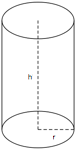## ASVAB Math Knowledge Practice Test 869658

Question 1 of 5
###### Cylinders

A cylinder is a solid figure with straight parallel sides and a circular or oval cross section with a radius (r) and a height (h). The volume of a cylinder is π r2h and the surface area is 2(π r2) + 2π rh.The dimensions of this cylinder are height (h) = 6 and radius (r) = 2. What is the surface area?

 28π 80π 32π 120π